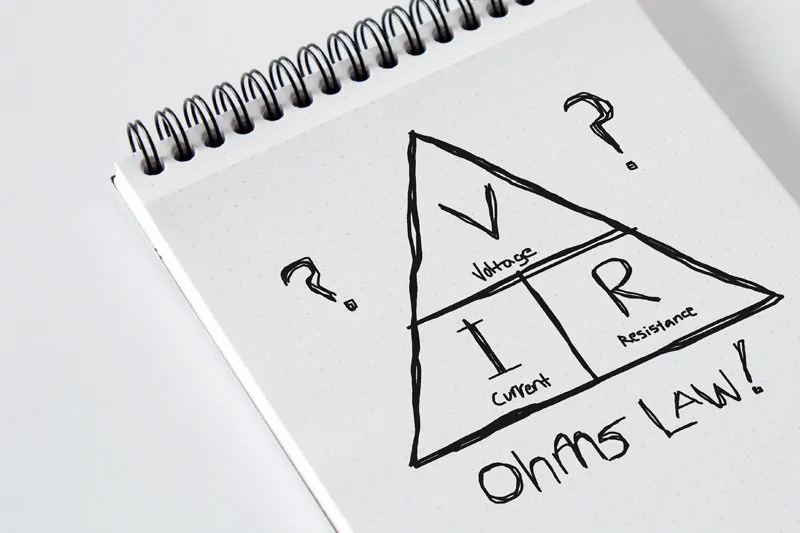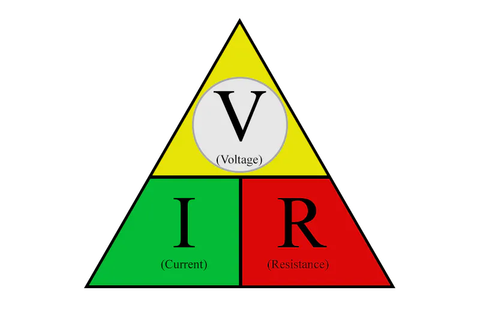If you are comfortable with your knowledge of vape battery safety, the next consideration is using some sort of calculator to make sure your coil builds are within that safe limits of your battery, and further, to allow you to tweak your coils to get the vaping experience you desire. There are tons of Ohm’s Law calculators, and sites like Steam Engine that will do the heavy lifting for you.

If you are happy with those and wish to remain blissfully ignorant about what is actually behind the calculations, good for you. As long as you know how to apply the results, you will live a long, happy, and safe vaping life. But if you want to see the inner workings of those calculators, read on.

There’s nothing mystical or magical about Ohm’s Law. It’s a few formulas, usually depicted inside of a triangle, and anyone can easily learn and use the formulas with any regular calculator.

The goal here is to show you the formulas behind Ohm’s Law and hopefully give you an understanding of the relationships between the different elements in a basic electronic circuit as related to vaping.

The TriangleInside the triangle you can see the three main elements in any electrical circuit, represented by the letters V, I, and R. I would vocalize the triangle as “V over I times R” with “times” being multiplication. The hardest part of this will be remembering what the letters represent, and even that is actually pretty easy:

·V = Voltage (your battery voltage)
·I = Current (the amperage drawn by your coil)
·R = Resistance (the resistance, in ohms, of your coil)

So, how do we use the Ohm’s Law triangle? Again, simple—the triangle visually depicts the relationship between voltage, current, and resistance. In the following examples, we’ll explore how to use the triangle and formulas to help you build coils targeting the current and wattage you desire.

Calculating currentIf you want to determine the current draw through a resistance (your coil) the formula is:

I = V ÷ R (or I = V/R)

How did we arrive at that? Look at the triangle and you will see that to solve for current (I) you must divide voltage (V) by resistance (R).

Let’s put the formula to work in a real-life example. If you are using a mechanical mod, with a freshly charged battery you theoretically have 4.2 V available to power your coil. If your coil is 0.5Ω, you now have everything you need to determine current, in amps:

I = 4.2 V ÷ 0.5Ω (or 4.2/0.5)

I = 8.4 A

As you can see, with your 0.5-ohm coil and a freshly charged battery at 4.2 volts, the resulting max current draw will be 8.4 amps. If your battery has a 10-amp limit, you are well below the cap. Don’t forget that using a dual mechanical mod in series configuration will double your amp draw per battery, and you will have to build coils with twice as high resistance to be safe. Also note that as the battery depletes, the current will also tail off. For example, when the battery reaches 3.7 volts with the same load, the current will drop to 7.4 amps (3.7 volts / 0.5 ohms)

Calculating power (wattage)
The next thing you will probably want to know is the power generated at the coil, or wattage. It’s not shown in the triangle, but the formula is simple. Just multiply the current in your circuit by the voltage applied:

P = V x I

In our original example, the formula would look like this:

P = 4.2 V x 8.4 A

P = 35.3 W

So that 0.5-ohm coil with a fully charged battery at 4.2 volts will pull a maximum of 8.4 amps and deliver 35.3 watts. You can see that as the resistance of your coil increases, current and wattage will both drop.

Calculating resistanceThe second Ohm’s Law formula that can be of use to us is calculating resistance. Let’s say that you have a battery with a 10-amp current limit and you want to determine the lowest coil resistance that you can safely run without exceeding the CDR of the battery.

To calculate, you would use the following formula:

R = V ÷ I

Since you know that the battery CDR is 10 amps, you might want to target 9 amps in your calculation, to give yourself 1 amp of headroom. You also know that your max voltage will be 4.2 volts on a single battery mod. So the calculation goes like this:

R = 4.2 V ÷ 9 A

R = 0.47Ω

The result tells you that your safe lower limit with the 10-amp battery is 0.47 ohms—anything lower and you risk exceeding the current limit of the battery. Of course, if you have a 25-amp battery, your low resistance drops to 0.17 ohms:

R = 4.2 V ÷ 25 A

R = 0.17Ω

Calculating voltageFinally, and probably not as useful to us, using the triangle you can solve for voltage in a circuit, as long as you know the values of the other two variables.

To solve for voltage when current and resistance is known, the formula looks like this:

V = I x R

What does it all mean?
Really, the most useful formulas for vapers, are the three that calculate current (I = V ÷ R) power (P = V x I) and resistance (R = V ÷ I). These will allow you to figure out the current your coil will draw and the wattage that will result. As you increase resistance, current and power will drop off. If you decrease resistance, current and power will increase. The resistance formula allows you to calculate a safe low resistance based on the CDR of your battery.

It’s all good information to help you stay within the safe limits of your batteries and to tweak the amount of power at your coil to help you achieve your own vaping nirvana. There are other considerations like coil ramp time and the heat of your coil that are determined by wire gauge and mass. Ohm’s Law won’t figure any of that, and a site like Steam Engine can be helpful.

One final, and critical piece of advice: ALWAYS assume that your battery voltage is the equivalent of a fully charged battery: 4.2 volts for a single battery mod or parallel battery mod, or 8.4 volts for a dual series mod. People will argue that the coil will never see that actual battery voltage due to voltage drop within the mod, but to be safe, ALWAYS use the full theoretical battery voltage (at full charge) in your calculations.